# 3. The flow net shown below illustrates flow conditions beneath a concrete dam featuring an upstream...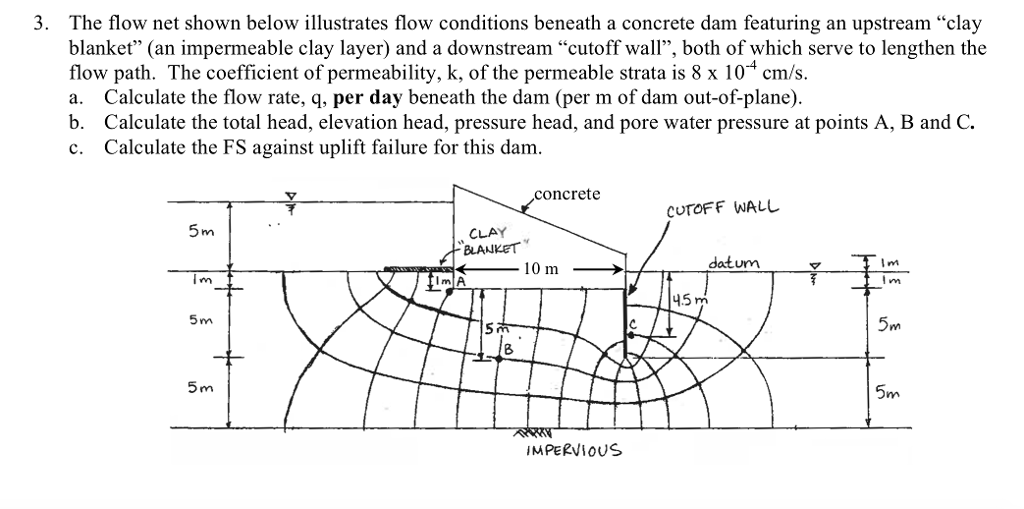3. The flow net shown below illustrates flow conditions beneath a concrete dam featuring an upstream "clay blanket" (an impermeable clay layer) and a downstream "cutoff wall", both of which serve to lengthen the flow path. The coefficient of permeability, k, of the permeable strata is 8 x 10 cm/s. a. Calculate the flow rate, q, per day beneath the dam (per m of dam out-of-plane). b. Calculate the total head, elevation head, pressure head, and pore water pressure at points A, B and C. c. Calculate the FS against uplift failure for this dam. concrete CUTOFF WALL 5m CLAY datum il 4.5 m 5m 5m 8 5m 5m IMPERViOUS

a, The flow rate is given by q = k x H x Nf / Nd

k = 8 x 10-4 cm/sec

H = 5-1= 4 m

Nf = 4

Nd = 14

q = 8 x 10-4 x 10-3 x 4 x 4/14 = 9.143 x 10-7 m2/sec per metre of dam out of plane

= 9.143 x 10-7 x 3600 x 24 = 0.0789942 m2/day per metre out of dam out of plane

b.

Nf = 14

Head drop = 16/14 = 1.143m per equipotential drop;

Head at point A = 16- 3x1.143 = 12.571

Head at point B=16- 5x1.143 = 10.258

Head at point C= 16-9x1.143= 5.713

c.

FS against uplift faillure for this dam= icritical/iactual ;

icritical = (G-1)/(1+e) = (2.7-1)/(1+0.85) = 0.865

iactual =  4/(11x1) = 0.3636

FS against uplift failure = 0.865/0.3636 = 2.379

#### Earn Coin

Coins can be redeemed for fabulous gifts.

Similar Homework Help Questions
• ### 5 8 7 8 9 10 111 112 113 114 15 Q3b(i) Figure shows a flow...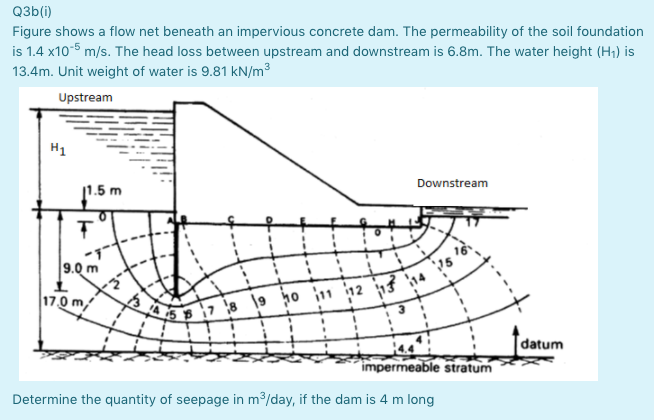5 8 7 8 9 10 111 112 113 114 15 Q3b(i) Figure shows a flow net beneath an impervious concrete dam. The permeability of the soil foundation is 1.4 x10-6 m/s. The head loss between upstream and downstream is 6.8m. The water height (H1) is 13.4m. Unit weight of water is 9.81 kN/m3 Upstream H1 11.5 m Downstream T 9.0 m 17.0 m; datum impermeable stratum Determine the quantity of seepage in m/day, if the dam is 4 m...

• ### *need the uplift force Problem 5 Consider the flow net shown below. The sandy soil has...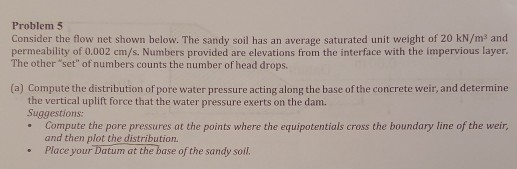*need the uplift force Problem 5 Consider the flow net shown below. The sandy soil has an average saturated unit weight of 20 kN/m and permeability of 0.002 cm/s. Numbers provided are elevations from the interface with the impervious layer. The other "set" of numbers counts the number of head drops. (a) Compute the distribution of pore water pressure acting along the base of the concrete weir, and determine the vertical uplift force that the water pressure exerts on the...

• ### Q.3. A flow net is shown below for a concrete spillway. Assume all elements of the...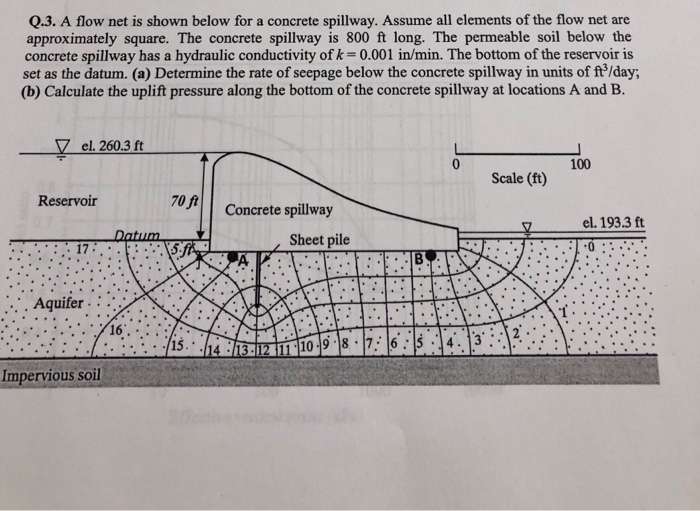Q.3. A flow net is shown below for a concrete spillway. Assume all elements of the flow net are approximately square. The concrete spillway is 800 ft long. The permeable soil below the concrete spillway has a hydraulic conductivity of k -0.001 in/min. The bottom of the reservoir is set as the datum. (a) Determine the rate of seepage below the concrete spillway in units of ft/day; (b) Calculate the uplift pressure along the bottom of the concrete spillway at...

• ### 2. Complete the following for the impermeable dam shown below: a. Identify a datum, indicate the...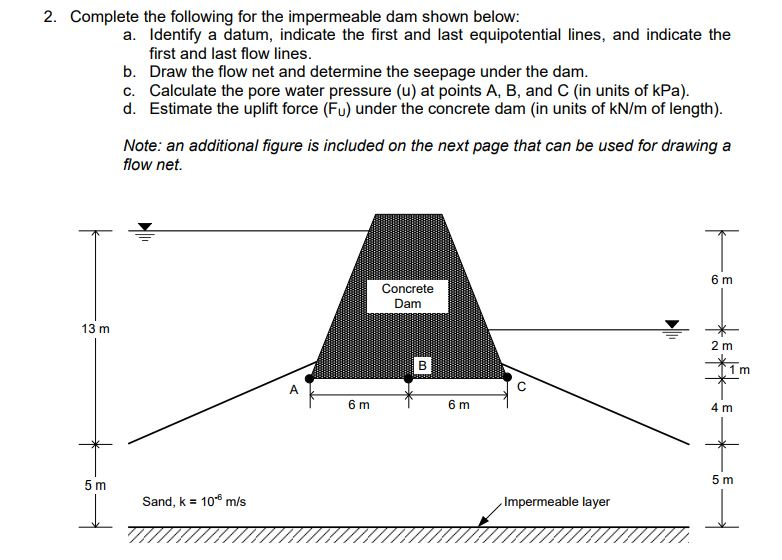2. Complete the following for the impermeable dam shown below: a. Identify a datum, indicate the first and last equipotential lines, and indicate the first and last flow lines b. Draw the flow net and determine the seepage under the dam. Calculate the pore water pressure (u) at points A, B, and C (in units of kPa). d. c. Estimate the uplift force (Fu) under the concrete dam (in units of kN/m of length) Note: an additional figure is included...

• ### 10. Calculate the following parameters from the flow net below. (a) The seepage under the dam...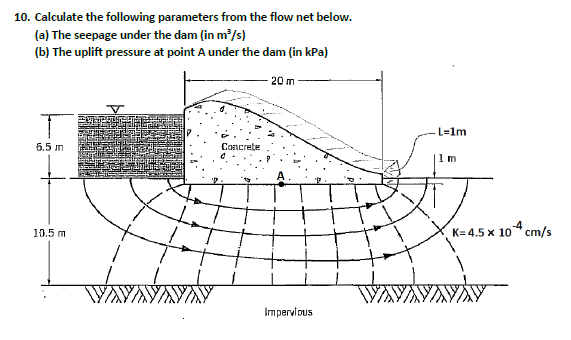10. Calculate the following parameters from the flow net below. (a) The seepage under the dam (in m/s) (b) The uplift pressure at point A under the dam (in kPa) 20 m L-1m 6.5 m Concrete 1 m K4.5 x 10 cm/s 10.5 m AYANAYAYA Impervious

• ### Problem (2) (12) For the concrete dam and soil profile shown in the Figure below, the...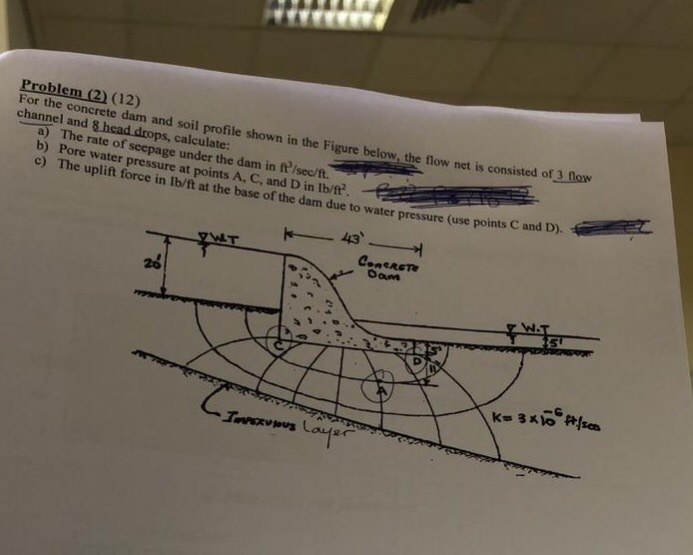Problem (2) (12) For the concrete dam and soil profile shown in the Figure below, the flow net is cons channel and 8 isted of 3 fNow rops, calculate: a) The rate of seepage under the dam in ft /sec/ft. b) Pore water pressure at points A, C, and D in Ib/ft. c) The uplift force in lb/ft at the base of the dam due to water pressure C use points C and D). 20

• ### 50 m Impermeable Concrete dam 6 m 1 m 10 m PtA (at mid-point of layer...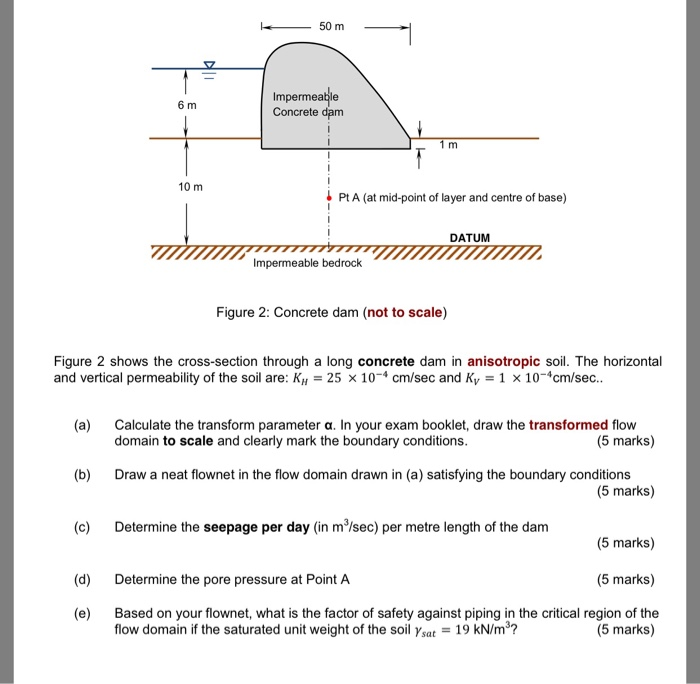50 m Impermeable Concrete dam 6 m 1 m 10 m PtA (at mid-point of layer and centre of base) DATUM Impermeable bedrock Figure 2: Concrete dam (not to scale) Figure 2 shows the cross-section through a long concrete dam in anisotropic soil. The horizontal and vertical permeability of the soil are: K 25 x 10-4 cm/sec and Ky 1 x 10-cm/sec.. (a) Calculate the transform parameter a. In your exam booklet, draw the transformed flow domain to scale and...

• ### Draw a flow netlfor the case of a long concrete dam shown in the Figure below...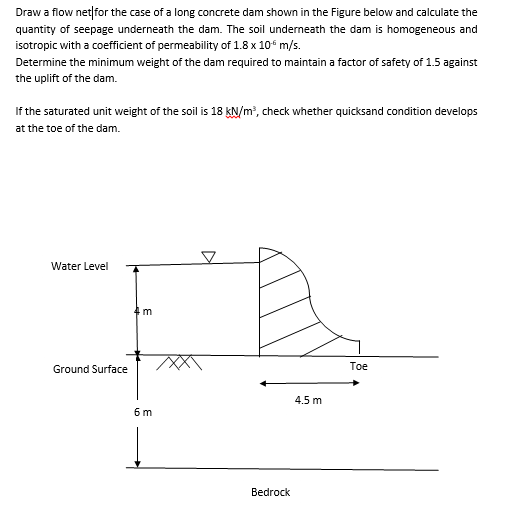Draw a flow netlfor the case of a long concrete dam shown in the Figure below and calculate the quantity of seepage underneath the dam. The soil underneath the dam is homogeneous and isotropic with a coefficient of permeability of 1.8 x 106 m/s. Determine the minimum weight of the dam required to maintain a factor of safety of 1.5 against the uplift of the dam. If the saturated unit weight of the soil is 18 KN/mP, check whether quicksand...